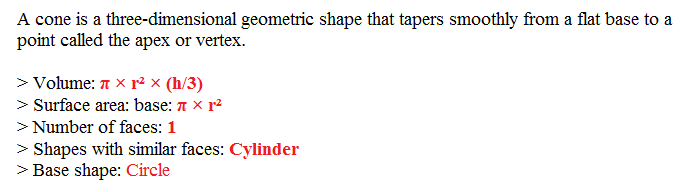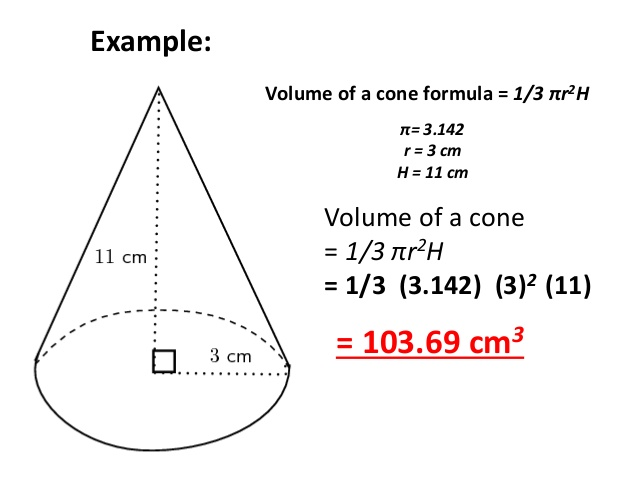# Volume Of Cone Java Program In 4 Simple Ways | Programs

Calculate the volume of the cone java program. There may be so many ways to find out the volume of a cone in Java; we are here to share the simple method. We also added the online execution & compile tool at the end of each program.

Check it out. If you have any doubts related to this section, then make a comment at the end of the post our dedicated expert team in Java development will glad to help you out related to the calculation of cone volume in Java.

1. Example -1: Standard Java program to calculate the volume.
2. Example -2: Java program with the help of command line arguments.
3. Example – 3: Java program by using the return method.

Before we get into the topic a couple of lines about what is cone?

What is a cone?The Formula to find out the volume of a cone?Example program # 1: To determine the volume of a cone by using the formula.

Output :

# Online Execution for the above program example -1 here check it out #

A step by step explanation of the above code. If you knew the basics of Java programming, then skip the following tutorial and move on to the next example program #2 and example program #3.

– The method gives the necessary values for the scanner function.

-The class is a blueprint and creates objects within a class.

– the Main function, the program starts reading the values from the primary function.

– Where scanner can read the input values with the help of the import java.util.Scanner; whereas system.in can read the values from your system of or device.

– Output displayed on the screen as per user requirements.

– Syntax to store the values in a particular format here ( Double ). Same follows for the rest of code lines too.

The formula to find out the volume of a cone here.

Output displayed here.

So you got an idea how the java program works to find the volume of a cone. Here is another example method for you. Here we are using the command line argument.

Example # 2 ( Using command line arguments with online execution tool )

Online Execution And Compile Tool for the above java program:

The difference between the standard java program and with the help of command line arguments are nothing but a small change in syntax. Where is in command line arguments using “ Parse ” are giving the input values before the program executes.

Whereas in example #1, we are providing the data values according to the program execution method. If you need to know more information about the parse method;

Here we share the complete guide about parse checks it out. Still, if you require knowing any more details about the calculation of a volume of cone Java program, then you can comment here at the end of the post we are glad to help you out.

Example Method # 3: Using invoked return method ( If you are a newbie to learn the java program then we kindly suggest you check out the above two examples only, for advanced users see the below program )

Here we go # Example: 3

That’s the complete example to calculate the volume ofthe cone java program. If you have any doubts related to above examples, do comment here. Our expert authors will help you out.

x

## X Star Pattern Java Program – Patterns

Java program to print X star pattern program – We have written the below print/draw ...